Question

What length of column would be required to just resolve two peaks with retention times of 92 and 107 s, respectively, using a column with an Hof 1.60 cm/plate?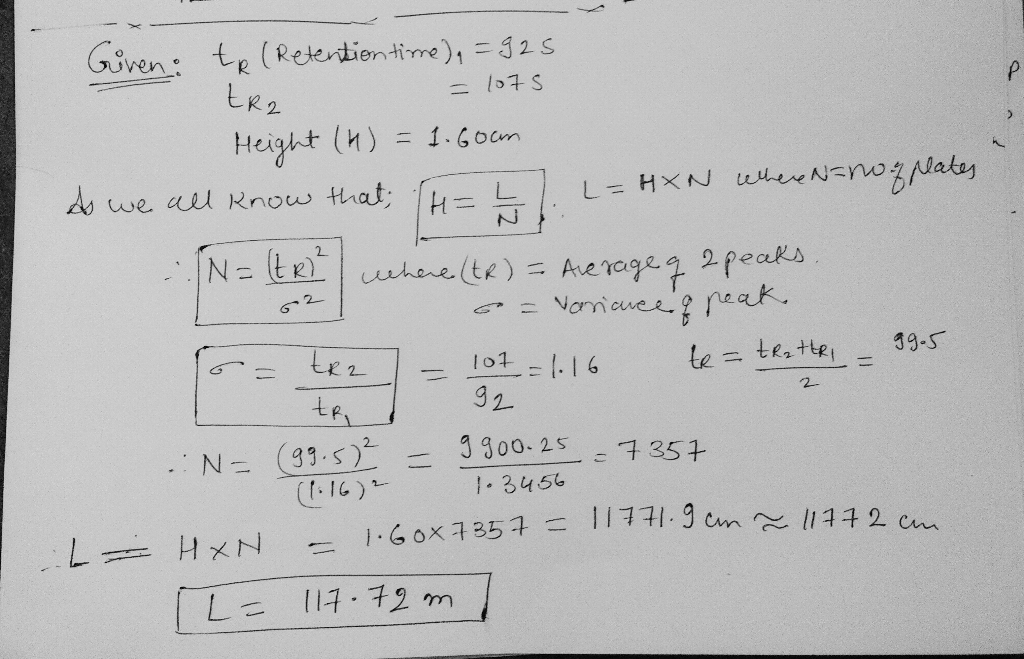#### Earn Coins

Coins can be redeemed for fabulous gifts.

Similar Homework Help Questions
• ### 4. The compounds in a mixture separated by GC can be identified by their retention times....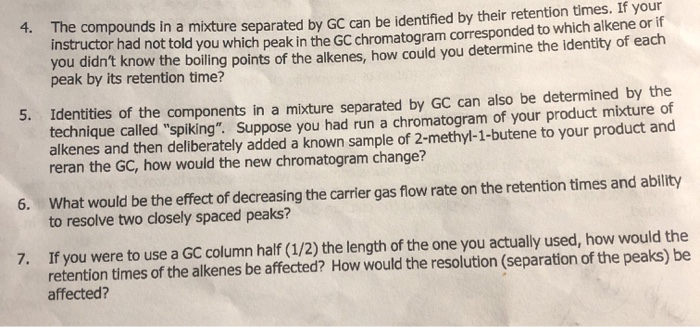4. The compounds in a mixture separated by GC can be identified by their retention times. If you Suructor nad not told you which peak in the GC chromatogram corresponded to which alker you didn't know the boiling points of the alkenes, how could you determine the identity or each peak by its retention time? Identities of the components in a mixture separated by GC can also be determined by the technique called "spiking". Suppose you had run a chromatogram...

• ### What would happen to the retention time of a compound if the following changes were made?...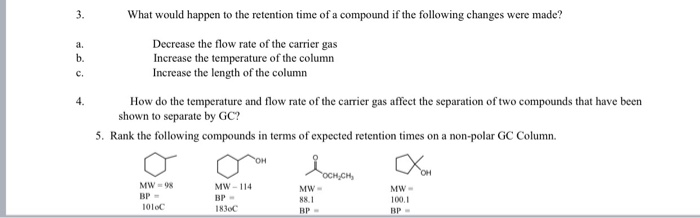What would happen to the retention time of a compound if the following changes were made? Decrease the flow rate of the carrier gas Increase the temperature of the column Increase the length of the column How do the temperature and flow rate of the carrier gas affect the separation of two compounds that have been shown to separate by GC? 5. Rank the following compounds in terms of expected retention times on a non-polar GC Column TOH OCH.CH MW-114...

• ### (3) During the separation of two steroids by HPLC on a C18 column, a student reported the following elution times with...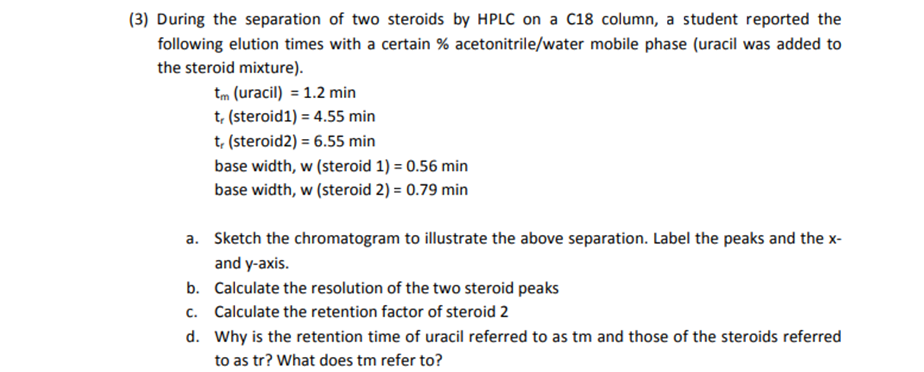(3) During the separation of two steroids by HPLC on a C18 column, a student reported the following elution times with a certain % acetonitrile/water mobile phase (uracil was added to the steroid mixture) tm (uracil) 1.2 min t, (steroid1) 4.55 min t, (steroid2) 6.55 min base width, w (steroid 1) 0.56 min base width, w (steroid 2) 0.79 min a. Sketch the chromatogram to illustrate the above separation. Label the peaks and the x- and y-axis. Calculate the resolution...

• ### A peak elutes from an HPLC column 17.3 cm in length in 14.0 min. What would...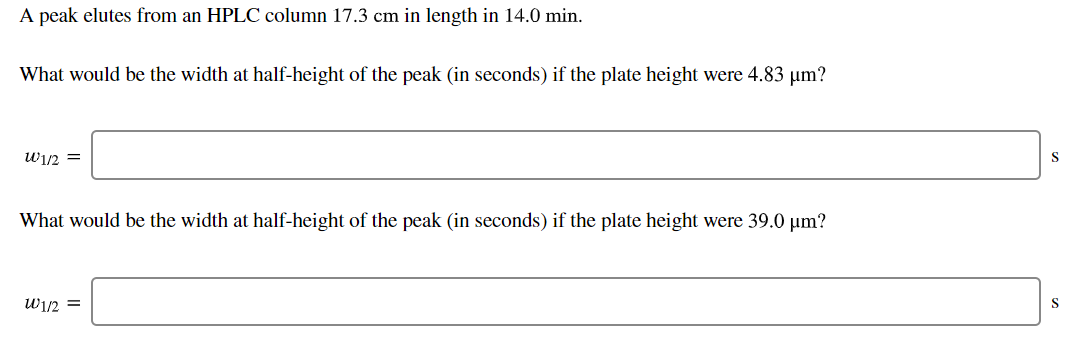A peak elutes from an HPLC column 17.3 cm in length in 14.0 min. What would be the width at half-height of the peak (in seconds) if the plate height were 4.83 um? W1/2 = What would be the width at half-height of the peak (in seconds) if the plate height were 39.0 um? W1/2 =

• ### A peak elutes from an HPLC column 11.8-cm in length in 13.8 min. What would be...

A peak elutes from an HPLC column 11.8-cm in length in 13.8 min. What would be the width at half-height of the peak (in seconds) if the plate height were: (a) 6.9 μm? (b) 36 μm?

• ### need help with number 2 HW #3 for Chem 318 Due: April 29, 2019 1. Solute A has a K 2.5 for an extraction between water (phase 1) and chloroform (phase 2). If 100 mL of a 0.01 fraction will be extr...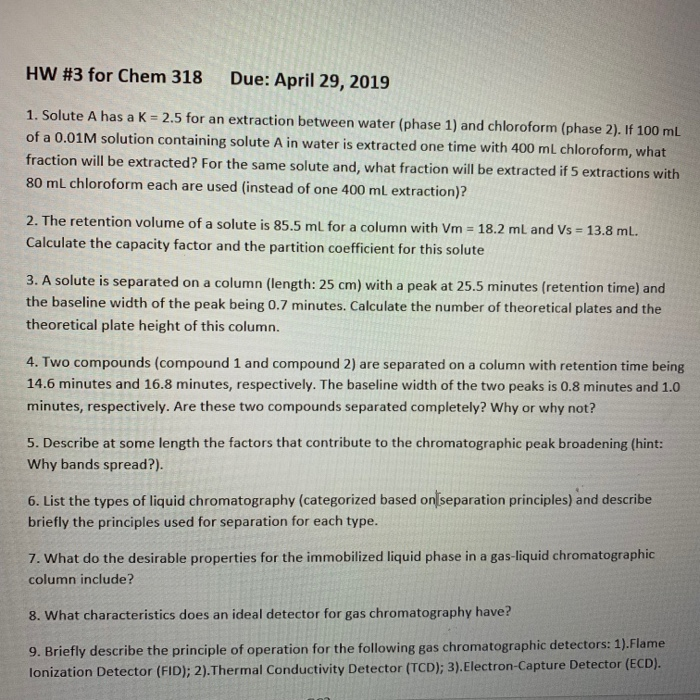need help with number 2 HW #3 for Chem 318 Due: April 29, 2019 1. Solute A has a K 2.5 for an extraction between water (phase 1) and chloroform (phase 2). If 100 mL of a 0.01 fraction will be extracted? For the same solute and, what fraction will be extracted if 5 extractions wi 80 mL chloroform each are used (instead of one 400 ml extraction)? M solution containing solute A in water is extracted one time with...

• ### A number of factors determine the rate at which a given compound travels through a gas chromatograph. List two of t...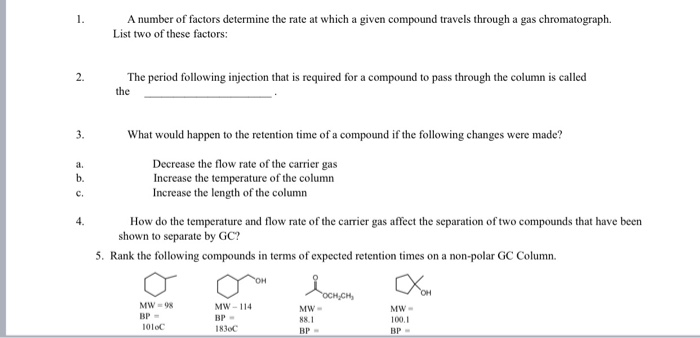A number of factors determine the rate at which a given compound travels through a gas chromatograph. List two of these factors: The period following injection that is required for a compound to pass through the column is called the What would happen to the retention time of a compound if the following changes were made? Decrease the flow rate of the carrier gas Increase the temperature of the column Increase the length of the column How do the temperature...

• ### 9) For the air wind tunnel shown above in Q8: (6 points) Assuming a circular cross...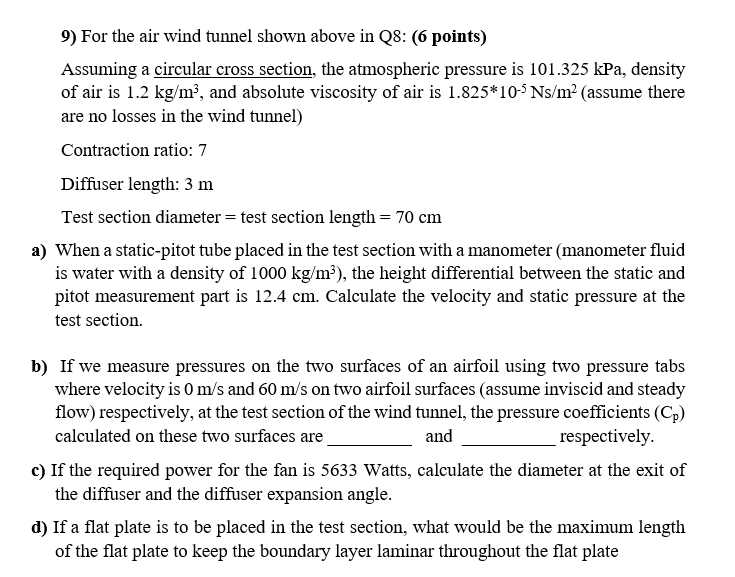9) For the air wind tunnel shown above in Q8: (6 points) Assuming a circular cross section, the atmospheric pressure is 101.325 kPa, density of air is 1.2 kg/m², and absolute viscosity of air is 1.825*10-Ns/m² (assume there are no losses in the wind tunnel) Contraction ratio: 7 Diffuser length: 3 m Test section diameter = test section length = 70 cm a) When a static-pitot tube placed in the test section with a manometer (manometer fluid is water with...

• ### Honeycomb Flow Straightener - Anti-Turbulence Screens Silencer Fan Test Section Diffuser Iniet Assuming a circular cross...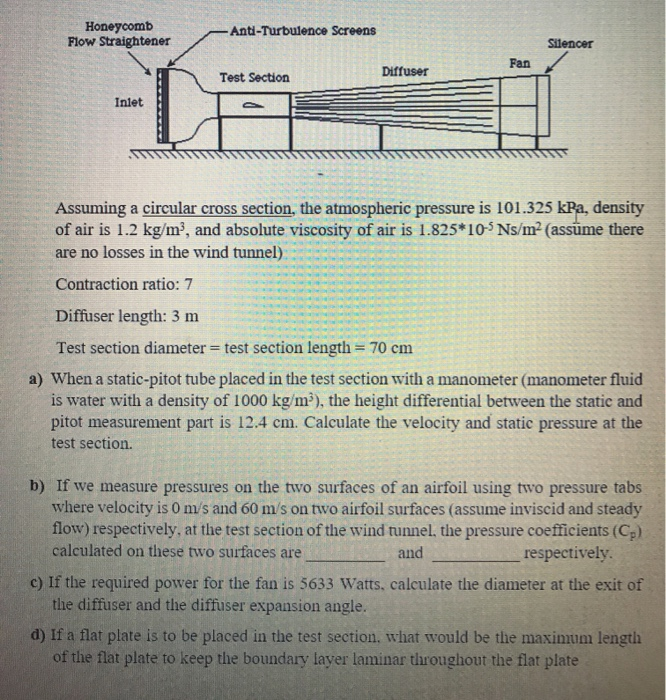Honeycomb Flow Straightener - Anti-Turbulence Screens Silencer Fan Test Section Diffuser Iniet Assuming a circular cross section, the atmospheric pressure is 101.325 kPa, density of air is 1.2 kg/m², and absolute viscosity of air is 1.825*10% Ns/m? (assume there are no losses in the wind tunnel) Contraction ratio: 7 Diffuser length: 3 m Test section diameter = test section length = 70 cm a) When a static-pitot tube placed in the test section with a manometer (manometer fluid is water...

• ### For the air wind tunnel shown Honeycomb Flow Straightener Anti-Turbulence Screens Silencer Fan Diffuser Test Section...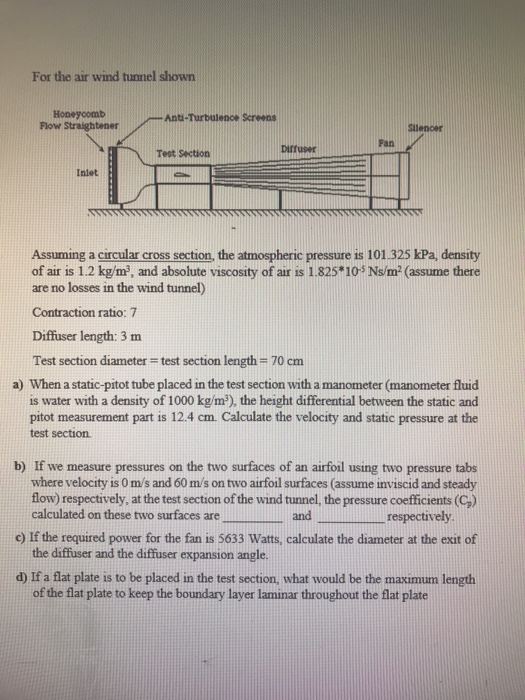For the air wind tunnel shown Honeycomb Flow Straightener Anti-Turbulence Screens Silencer Fan Diffuser Test Section Inlet Assuming a circular cross section, the atmospheric pressure is 101.325 kPa, density of air is 1.2 kg/m?, and absolute viscosity of air is 1.825*10-S Ns/m? (assume there are no losses in the wind tunnel) Contraction ratio: 7 Diffuser length: 3 m Test section diameter = test section length = 70 cm a) When a static-pitot tube placed in the test section with a...